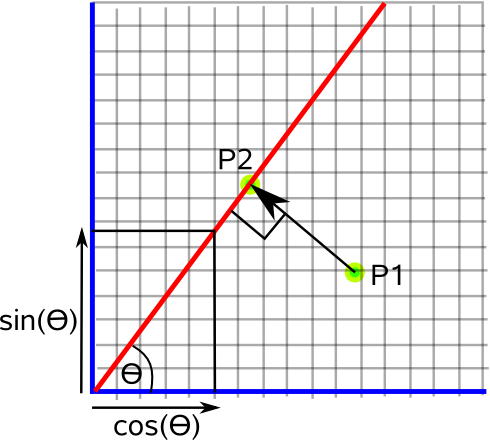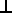# Maths - Projections

This page explains various projections, for instance if we are working in two dimensional space we can calculate:

• The component of the point, in 2D, that is parallel to the line.
• The component of the point, in 2D, that is perpendicular to the line.
• A mapping from the 2D point to one dimensional space represented by the line.
• A mapping from the one dimensional distance along the line to the position in 2 space.

These transformations are related as we will discuss.

The following pages extend this topic:

To start with the simplest case consider a point projected onto a two dimensional line.If we want to map the two dimensional space shown here onto the one dimensional space of the line we can use:

P2 = line • P1

where:

• P1 = vector representing a point to be transformed
• P2 = scalar representing point in terms of its distance along line.
• • = vector dot product.
• line = line represented by a normalised length vector (cos(θ),sin(θ)).

In matrix or tensor terms this would be:

P2 =
 cos(θ) sin(θ)
 P1x P1y

In other words we transpose the line vector and multiply it by the vector represented by the point in question.

In complex number terms this would be:

P2 = real(line' * P1)

where:

• P1 = point to be transformed P1x + i P1y
• P2 = scalar representing point in terms of its distance along line.
• real() = a function which takes the real part and sets the imaginary part to zero .
• line = cos(θ) + i sin(θ)
• line' = cos(θ) - i sin(θ)

## One dimension to a line in two dimensions

We can also do the inverse transform, that is, take an input which is a scalar distance along a line and to map this to a two dimensional position on the line.

P2 =
 cos(θ) sin(θ)
* P1

where:

• P1 = scalar input representing a distance along the line
• P2 = vector representing point on the line.

# Result left in 2 dimensions

What if we want to map the points onto a line but leave that line in two dimensional space (as is shown in the diagram above)? We can do this by mapping to a scalar value and then mapping back to two dimensions, that is, apply both of the above transforms in sequence.

We will use the notation:

P2 = P1 || line

That is the component of P1 that is parallel to the line.

In matrix or tensor terms this would be given by:

P2 =
 cos(θ) sin(θ)
 cos(θ) sin(θ)
 P1x P1y

Where, in this case,

• P2 = vector giving the position of the point after it is transformed onto the line.

Multiplying the terms gives:

P2 =
 cos2(θ) sin(θ)*cos(θ) sin(θ)*cos(θ) sin2(θ)
 P1x P1y

We can also calculate the perpendicular distance of the point from the line

We will use the notation:

P2 = P1line

That is the perpendicular distance between P1 and the line.

this is given by:

P2 = (
 1 0 0 1
-
 cos2(θ) sin(θ)*cos(θ) sin(θ)*cos(θ) sin2(θ)
)
 P1x P1y

Which gives:

P2 =
 1 - cos2(θ) -sin(θ)*cos(θ) -sin(θ)*cos(θ) 1 - sin2(θ)
 P1x P1y

# Using Geometric Algebra Notation

We can repeat the above results using geometric algebra notation.

#### Mapping 2D to 1D

Mapping a point in 2 (or more) dimensions to a one dimensional (scalar) value to represent the distance along the line.

A first attempt at this would be A•B

where:

• A = vector representing a point.
• B = vector representing a line.

This gives a scalar as required, but it is not quite right unless B is unit length, otherwise we can use:

a = (A•B)/|B|

where:

• a = scalar length along line.
• A = vector representing a point.
• B = vector representing a line.

#### Mapping 1D to 2D

We can use scalar multiplication to get the two dimensional position from the scalar length along the line.

A = (a*B)/|B|

where:

• a = scalar length along line.
• A = vector representing a point.
• B = vector representing a line.

#### Parallel Component of a Point

We can calculate the parallel component of a point (A) to a line (B) by converting to a one dimensional distance along the line and that converting back to two dimensions. To do this we combine the above expressions as follows:

A || B = A•B * B/|B|2

#### Perpendicular Component of a Point

AB = (A x B) x B /|B|2

#### Summary

Since (B•B) = |B|2 we can rewrite these expressions as:

• A || B = A•B * B/(B•B)
• AB = (A x B) x B /(B•B)

# Higher Dimensions

We can also project onto a line in higher dimensions such as 3D, we just increase the dimension of the vectors that we are using.

• P1 = vector representing a point to be transformed, this will now be (P1x,P1y,P1z)
• line = line represented by a normalised length vector. This will now be (x,y,z) where x2+y2+z2=1.

# Other Projections

 metadata block see also: Correspondence about this page Book Shop - Further reading. Where I can, I have put links to Amazon for books that are relevant to the subject, click on the appropriate country flag to get more details of the book or to buy it from them.Applied Geometry for Computer Graphics...

This site may have errors. Don't use for critical systems.